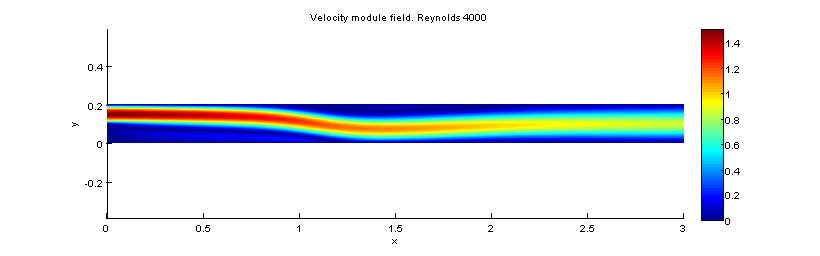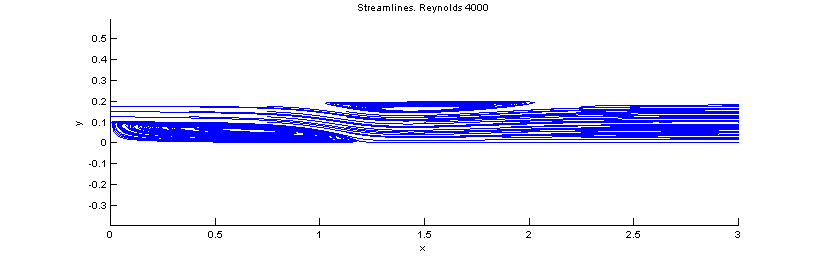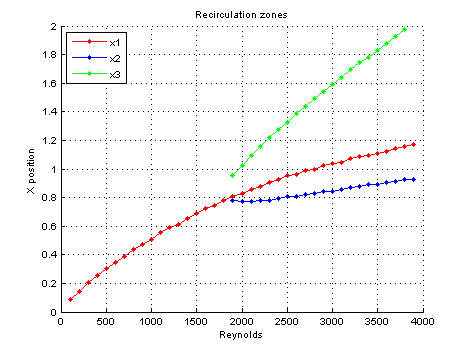# Simulation of a Backwards Facing Step

Coded by Paul Valcke and Luis Bernardos. Here we simulate the flow of a Backwards facing step. We solve the laminar steady Navier Stokes equations, by means of a Newton method using the Jacobian of the equation. Hence, the solved equation (appart from \partial_iu_i=0 of course) is:

\displaystyle u_j\partial_ju_i = -\partial_ip + \frac{1}{Re}\partial_{jj}^2u_i

The flow being modeled is also a very typical case of study in CFD. A stablished laminar Poiseuille’s flow enters through a channel, and encounters an abrupt change in section because of a step. Therefore, the boundary layer at this step will be detached, creating a recirculating vortex. Moreover, if the Reynolds number is big enough, other recirculating zones can be created within the flow (especially at the top wall). Through this code we intend to monitor the recirculating bubble(s) as a function of the Reynolds number. Indeed, this is a Steady code, so for calculating high Reynolds number solution we must have a good solution initialization. That’s why we use an increasing-Reynolds loop strategy, like we did in the Lid Driven Cavity flow.

clear all; figure('OuterPosition',[0, 0, 1000, 400]); format compact


# Parameters

Mostly self-explained parameters. This is a Steady calculation, so we implemented a simple strategy of increasing Reynolds, so that we can simulate relatively high Reynolds number re-using a lower reynold number as solution initialization.

%%%% parameters
Re=100; % Starting reynolds number
ReMax = 4000; % Stablished reynolds number
ReNum = 39; % amount of Reynolds steps
DiamInlet = 0.1; % Diameter of inlet
Uinlet = 1;
Lx = 3; % X Length of domain
Ly = 0.2; % Y Length of domain
Nx=250; % number of grid nodes in x
Ny=120; % number of grid nodes in y
pts=3; % number of points in finite difference stencils
alpha=1; % Under-relaxation. May help convergence.
maxIter = 200;
resSTOP = 1e-5;

ReStep = (ReMax-Re)/ReNum;

# Mesh

We want to shrink the mesh near the walls, for making a good calculation of the boundary layer. Therefore, we make use of Lspacing function, with a \lambda=1, which means cosinus discretization. We perform this only on the y direction. Then, we have to transform our differentiation matrices making use of map2D.

% differentiation
[d.x,d.xx,d.wx,x]=dif1D('fd',0,Lx,Nx,pts);
[d.y,d.yy,d.wy,y]=dif1D('fd',0,Ly,Ny,pts);
[D,l,~,~,Z,I,NN]=dif2D(d,x,y);
y = Lspacing(Ny,1,Ly); % Shrinks the mesh on the top and bottom walls.
[X,Y] = meshgrid(x,y);
D = map2D(X,Y,D);
D.lap=D.yy+D.xx;
%mesh(X,Y,0*X,'EdgeColor','k'); view(2); axis equal; grid off;break


# Boundary conditions

• Velocity-Inlet: Dirichlet condition, imposing Poiseuille’s flow.
• Wall: Dirichlet no-slip ondition, on the top and bottom wall, as well as the descending step.
• Outflow: Neumann on velocity conditions, at the right end of the mesh. Note that here the airflow should be established as a Poiseuille’s flow again.

We have to find the indices of the Velocity Inlet:

% Stores the indices of the inlet and its corresponding values
y_inlet_ind = find(Y(l.left)>=DiamInlet);
y_inlet = Y(y_inlet_ind);
%%%% preparing boundary conditions
u=(1:NN)'; v=u+NN; p=v+NN;
II=speye(3*NN);

% Conditions
dir=[l.ctl;l.ctr;l.left;l.top;l.bot]; % where to put Dirichlet on u and v
loc=[u(dir); v(dir); % Dirichlet
u(l.right);  % Neumann
v(l.right)]; % Neumann

C=[II([u(dir);v(dir)],:); % ...     % Dirichlet on u,v
D.x(l.right,:), Z(l.right,:), Z(l.right,:);   % Neuman on u at outflow
Z(l.right,:),  D.x(l.right,:),Z(l.right,:)];  % Neumann on v at outflow


We chose an initial guess that statisfies the boundary conditions, this is good for Newton, and it makes it also very easy to impose the nonhomogeneous boundary conditions in the lop.

% initial guess
U = zeros(NN,1);

Here is where we impose the Poiseuille’s flow at the inlet:

U(y_inlet_ind) = 1.5.*Uinlet.*(1-((2.*(y_inlet-min(y_inlet)))./DiamInlet - 1).^2); % Poiseuille
V=zeros(NN,1);
P=ones(NN,1);
q0=[U(:);V(:);P(:)];

% Newton iterations
x1=[]; x2=[]; x3=[]; Re1 = []; Re2 = []; Re3 = []; % Invokes monitoring variables
disp('Newton loop')
q=q0;
qM=q;
quit=0;count=0;
while ~quit

% the present solution and its derivatives
U=alpha.*q(u) + (1-alpha).*qM(u);
V=alpha.*q(v) + (1-alpha).*qM(v);
P=alpha.*q(p) + (1-alpha).*qM(p);
Ux=D.x*U; Uy=D.y*U;
Vx=D.x*V; Vy=D.y*V;
Px=D.x*P; Py=D.y*P;


This is now the heart of the code: the expression of the nonlinear fonction that should become zero, and just after, the expression of its Jacobian, then the boundary conditions.

    % nonlinear function
f=[-(U.*Ux+V.*Uy)+(D.lap*U)/Re-Px; ...
-(U.*Vx+V.*Vy)+(D.lap*V)/Re-Py; ...
D.x*U+D.y*V];

% Jacobian
A=[-( spd(Ux) + spd(U)*D.x + spd(V)*D.y )+(D.lap)/Re, -spd(Uy), -D.x; ...
-spd(Vx),  -(spd(Vy) + spd(V)*D.y + spd(U)*D.x )+(D.lap)/Re, -D.y; ...
D.x, D.y, Z];

% Boundary conditions
f(loc)=C*(q-q0);
A(loc,:)=C;

Plotting

    % plotting
Module = sqrt((U.^2+V.^2));
surf(X,Y,reshape(Module,Ny,Nx)); view(2); axis equal; shading interp; grid off; colorbar;
xlabel('x'); ylabel('y'); title(sprintf('Velocity module field. Reynolds %0.0f',Re));
drawnow

% convergence test
res=norm(f);
disp([num2str(count) '  ' num2str(res)]);
if (count>maxIter) || (res>1e5); disp('no convergence');break; end
if res<resSTOP && Re < ReMax
fprintf('converged for intermediate Re=%0.0f\n',Re);

# Monitoring

Here is where we monitor the solution. The first question is: what do we want to monitor? Well, it is true that there may exist several recirculation zones in the flow, but we will focus uniquely on the main top bubble and main down bubble (this is seen clearly through the streamlines plot). Therefore, we use the following variables:

• x_1: The “end” of the main recirculating bubble, right after the step, at the bottom wall.
• x_2: The “start” of the recirculating bubble located on the top wall.
• x_3: The “end” of the recirculating bubble located on the top of the wall.

Therefore, the length of the lower bubble is x_1, and the length of the higher bubble is x_3-x_2.

        x1_loc = find(U(l.bot+1)<0,1,'last');
x2_loc = find(U(l.top-1)<0,1,'first');
x3_loc = find(U(l.top-1)<0,1,'last');
if ~isempty(x1_loc), x1 = [x1; X(1,x1_loc)]; Re1=[Re1;Re];end
if ~isempty(x2_loc), x2 = [x2; X(1,x2_loc)]; Re2=[Re2;Re];end
if ~isempty(x3_loc), x3 = [x3; X(1,x3_loc)]; Re3=[Re3;Re];end
if ~isempty(x1),fprintf('x1 = %g ',x1(end));end
if ~isempty(x2),fprintf('x2 = %g ',x2(end));end
if ~isempty(x3),fprintf('x3 = %g ',x3(end));end
fprintf('\n');
if Re < ReMax, Re = Re + ReStep; end
elseif res<resSTOP && Re >= ReMax
quit=1;
fprintf('converged for Final Re=%0.0f\n',Re);
continue;
end

% Newton step
qM = q;
q=qM-A\f;
count=count+1;
end

# Plotting

% Saves the image of the velocity field
set(gcf,'paperpositionmode','auto')
print('-dpng','-r80','BackwardsFacingStep_Vel.png')

% Plots and saves the image of the vorticity field
Vorticity = -D.y*U + D.x*V;
surf(X,Y,reshape(Vorticity,Ny,Nx)); view(2); axis equal; shading interp; grid off; colorbar;
xlabel('x'); ylabel('y'); title(sprintf('Vorticity field. Reynolds %0.0f',Re));
caxis([-20 20]);
drawnow
set(gcf,'paperpositionmode','auto')
print('-dpng','-r80','BackwardsFacingStep_Vor.png')

% Plots and saves the image of the streamlines
[startx,starty] = meshgrid(0:Lx/8:Lx,0:Ly/8:Ly);
clf; streamline(X,Y,reshape(U,Ny,Nx),reshape(V,Ny,Nx),startx, starty);axis equal
xlabel('x'); ylabel('y'); title(sprintf('Streamlines. Reynolds %0.0f',Re));
drawnow
set(gcf,'paperpositionmode','auto')
print('-dpng','-r80','BackwardsFacingStep_SL.png')

% Plots the recirculating zones

It is important to note that there exists so-called “false positives”. This is a consequence of the way we use to calculate x_1, x_2 and x_3, that looks for a change in sign of the closest grid points of the wall. Therefore, here we delete those false positives.

Re2(x2>2) = [];x2(x2>2) = [];  Re3(x3>2) = []; x3(x3>2) = [];% Deletes false positives
figure;
hold on
plot(Re1,x1,'.-r','DisplayName','x1');
plot(Re2,x2,'.-b','DisplayName','x2');
plot(Re3,x3,'.-g','DisplayName','x3');
hold off
grid on;
xlabel('Reynolds'); ylabel('X position'); title('Recirculation zones');
legend('show','location','NorthWest');
set(gcf,'paperpositionmode','auto')
print('-dpng','-r80','BackwardsFacingStep_Rec.png')


# ResultsVelocity field for Reynolds 4000

The previous image shows the velocity module field at Reynolds 4000. We can remark that the flow is re-established as Poiseuille at the outflow.Vorticity fieldStreamlines

The streamlines here clearly shows the two recirculating laminar bubbles.Recirculating zones x_1, x_2 and x_3 as a function of the Reynolds number

The last image shows the recirculating zones as a function of the Reynolds number. We can see that the evolution of x_1 is almost linear, and we can also observe that the laminar top bubble only appears at Re>1800.